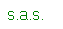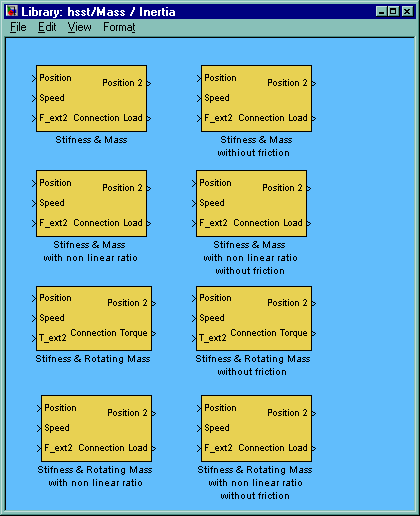Hyrdaulic Systems Simulation Tool

Mass / InertiaThere are eight blocks that represent moving masses connected by a flexible elements to the actuators.

As far as the second moving mass is concerned, it is also possible to have a reduction ratio between the mass and actuator. The transmission ratio is defined as the second mass speed over the first actuator speed; the second mass parameters (mass, conjunction stiffness, damping factor, position limits) shall be defined downstream the ratio. The output position is reported upstream to the transmission ratio.

More then one of these blocks can be connected in series.

There is also the possibility of having a non linear reduction ratio. The transmission ratio is defined as the ratio between downstream position over upstream position and is a function of the position. The relationship between ratio and position is defined by means of the 5 exponents of a 4 order polynomial that represents the trend of the ratio versus the actuator position expressed in meter:

Also for the second mass there are the blocks without friction.